# Overview of environmental airflow energy harvesting technology based on piezoelectric effect

## Huajie Zou1, Fuhai Cai2, Jianghua Zhang3, Zhenyu Chu4

1, 2, 3, 4Changzhou Vocational Institute of Mechatronic Technology, Jiangsu Changzhou, China

1Corresponding author

Journal of Vibroengineering, Vol. 24, Issue 1, 2022, p. 91-103. https://doi.org/10.21595/jve.2021.21979
Received 6 April 2021; received in revised form 24 September 2021; accepted 10 October 2021; published 26 November 2021

Copyright © 2021 Huajie Zou, et al. This is an open access article distributed under the Creative Commons Attribution License, which permits unrestricted use, distribution, and reproduction in any medium, provided the original work is properly cited.
Views 212
Abstract.

With the development of environmental energy harvesting technology, the research focus gradually develops from the energy harvester itself to the energy harvesting system of coupled environmental vibration sources, in which environmental airflow energy harvesting technology based on the piezoelectric effect is an important research direction. Here, the study status and development trend of piezoelectric energy harvesting were reviewed and summarized including rotation types, vortex-induced vibration types, flutter types and resonant types. It was found that the rotational multi-array energy harvesters are easy to scale, and the vortex-induced vibration and the flutter energy harvesters are poor adaptability for wide range of airflow velocity, and the resonant types can effectively improve excitation energy and output power. In order to improve power generation capacity and broaden the range of airflow velocity, energy harvesting with multiple frequency excitation and multi-piezoelectric array are future main development tendencies.

Highlights
• The development trend of piezoelectric energy harvesters were reviewed and summarized including rotation types, vortex-induced vibration types, flutter types and resonant types.
• Except for the resonant type, the other wind-induced vibration energy harvesters are suitable for low speed of the inflow and low working frequency.
• The piezoelectric energy harvesters are poor adaptability for wide range of the airflow velocity.
• To solve the contradiction between volume and energy caused by structure miniaturization, the cooperative energy exchange of multiple piezoelectric vibrators is an inevitable development trend.

Keywords: environmental energy, piezoelectric, energy harvesting, review.

#### 1. Introduction

Wireless sensor node network has been widely used in aerospace, military construction, transportation, agriculture and other fields , but the power supply is a new problem. Because of the disadvantages of short life, low power density and replaced regularly, the traditional battery power supply is limited . The energy of environmental airflow can be harvested and converted into electricity, which will be an effective way to supply. In recent years, environmental energy harvesting, such as wind energy, thermal energy and vibration energy, has attracted extensive attention from the domestic and foreign researchers. It can continuously convert environmental energy into electricity with the advantages of small volume, long life and high energy density. It has a widely application prospect. Within a variety of feasible viroelectric energy transfer systems, piezoelectric has the advantages of high energy output density, easily processed into various shapes or integrated with the matrix and conveniently miniaturized . It has been focused on the use of piezoelectric system to transform structural vibration into electricity.

Environmental airflow is a kind of green and sustainable energy with widely distributed, such as, wind, pipe flow and windstream of the moving object. The energy harvesting of the airflow excited piezoelectric energy harvester involves both the fluid field, solid field and electric field. It is a vibrational energy harvesting process with multiple physical fields coupled to each other, and it has high academic value and economic benefits.

Focusing on the energy harvesting types of environmental airflow, this paper has analyzed the research progress of rotation types, vortex-induced vibration types, flutter types and resonant types and summarized the structural characteristics and the applicable conditions of various piezoelectric energy harvesting. The future development trends also have been explored.

#### 2.1. The direct piezoelectric effect

The direct piezoelectric effect means that when the force applied to a certain direction of the piezoelectric crystal causes deformation, the surface of the piezoelectric crystal will produce the same amount of charge which is proportional to the magnitude of the force. And when the force disappears, the charge also disappears. When the direct piezoelectric effect occurs, the relation between the electric displacement vector $D$ and the stress $T$ or strain $S$ can be expressed as:

(1)

where, $d$ is the piezoelectric strain constant, $e$ is the piezoelectric stress constant.

Common piezoelectric materials used for energy harvesting include the polymer PVDF and the piezoelectric ceramic PZT. Piezoelectric ceramic has a large rigid shape, variable dielectric constant and high sensitivity. Polymer has high flexibility, low density, low impedance and good chemical stability.

#### 2.2. Piezoelectric structure types of the energy harvester

According to the different working modes of piezoelectric materials, the piezoelectric energy harvester usually has two types: cantilever beam and membrane, shown in Fig. 1. The piezoelectric cantilever type usually works in d31 mode. In the vibration process, strain is generated in the vertical direction when the deformation force is applied, and the charge generated in the horizontal direction is collected to realize the conversion from vibration energy to electric energy. The advantages of the cantilever type are simple structure and good energy transfer.

The piezoelectric membrane type usually works in d33 mode. In the vibration process, strain is generated in the vertical direction when deformation occurs when the force is applied, and the charge generated in the thickness direction is collected to realize the conversion from vibration energy to electric energy. The advantages of the membrane type are large natural frequency and high maximum output energy.

Fig. 1. Piezoelectric structure types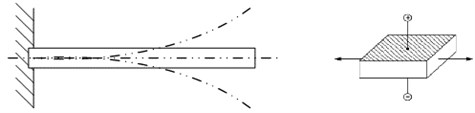a) Cantilever type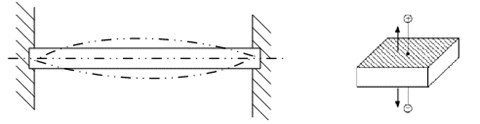b) Membrane type

#### 3. Classification of wind energy harvesters based on piezoelectric effect

In terms of the action of airflow excitation, it can be divided into static action and dynamic action. Static action mainly causes the rotation of the structure, while dynamic action mainly causes the vibration of the structure by unsteady aerodynamics.

#### 3.1. Piezoelectric energy harvester with rotation type

The piezoelectric energy harvesters with rotation type use blades to convert wind energy into rotational kinetic energy, which is converted into electricity by rotating blades that vibrate an array of piezoelectric beams, and finally the mechanical kinetic energy is converted into electrical energy. The resultant force acting on the blade can be expressed as follows:

(2)
$F=\frac{1}{2}\rho {C}_{r}S{V}^{2},$

where $\rho$ is the density of air, $S$ is the area of the blade, ${C}_{r}$ is the aerodynamic coefficient, $V$ is the speed of the incoming airflow.

Parameters of piezoelectric energy harvester with rotation type are shown in Table 1. Priya proposed a piezoelectric windmill with 12 piezoelectric bimorphs evenly fabricated around the circumference [4, 5], shown in Fig. 2. A power of 7.5 mW had been successfully measured across a load of 6.7 kΩ under wind velocity of 4.47 m/s. It can meet the demand of micro wireless sensor power supply. Myers proposed an optimized small scale piezoelectric windmill , shown in Fig. 3. The whole structure of the windmill is made of plastic, and it utilizes 18 piezoelectric bimorphs which makes this design extremely cost effective. The windmill was tested at average wind speed of 2.8 m/s and it provided 5 mW continuous power. Chang presented a portable power source driven by natural wind power, which can convert the constant wind power to AC-like wind power using a propeller and than electrical energy can be generated by PVDF vibration . It was found that maximum output voltage can reach 4.05 V with the wind speed of 3.5 m/s. Chan Ho Yang proposed a small windmill-type piezoelectric energy harvester . When the wind speed is 1.94 m/s, the working frequency of 10.25 Hz and the maximum output power of 3.14 mW were generated.

The problems of rotation type are that repeated friction between moving parts can cause parts to fail, producing noise while working, the wiring is complicated and the structure size is too large.

Fig. 2. Piezoelectric windmill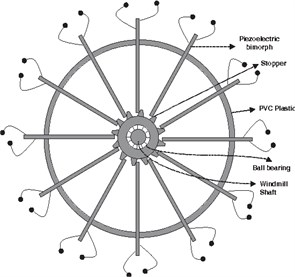Fig. 3. Small scale windmill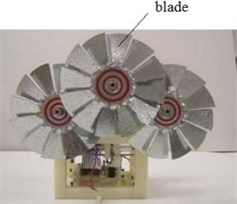a) The prototype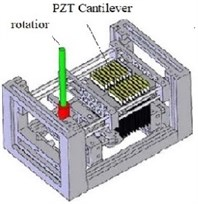b) The schematic

Table 1. Parameters of piezoelectric energy harvester with rotation type

 Researcher Air damper Piezoelectricmaterial Number Vibration frequency(Hz) Airflow velocity(m/s) Outputpower Matching resistance(kΩ) Powerdensity(mw/cm3) Priya blades PZTcantilever 12 65 4.47 7.5 mW 6.7 0.87 Myers blades PZTcantilever 18 4.5 2.8 5 mW 20 6.94 Chang blades PVDFcantilever 1 42.72 3.5 4.05 V – – Yang blades PZTcantilever 1 10.25 1.94 3.14 mW – 12.4

#### 3.2. Piezoelectric energy harvester with vortex-induced vibration type

Vortex-induced vibration is a common fluid-structure coupling phenomenon. Within a certain range of Reynolds Number, when the steady flow bypasses a non-streamlined blunt body, vortex shedding can be generated behind the blunt body on both sides. Meanwhile, vibration source can be formed by oscillation pressure with vortex shedding. The frequency of vortex shedding is related to the shape and size of blunt body and Strouhal number of the wind speed in the flow field, and the relationship can be expressed as:

(3)
${f}_{s}=\frac{{S}_{r}V}{D},$

where ${f}_{s}$ is the frequency of vortex shedding, ${S}_{r}$ is the dimensionless Strauhal number, $V$ is the wind speed, $D$ is characteristic dimensions of the blunt body.

The periodic pressure by vortex induced vibration acting on the blunt body has the same form as the harmonic force, the pressure is a harmonic force proportional to the lift coefficient. The equation of vortex induced vibration can be expressed as :

(4)
$m\left(\stackrel{¨}{y}+2\xi {\omega }_{n}\stackrel{˙}{y}+{\omega }_{n}^{2}y\right)=\frac{1}{2}\rho {V}^{2}B{C}_{L}\mathrm{s}\mathrm{i}\mathrm{n}\left({\omega }_{s}t+\phi \right),$

where ${C}_{L}$ is the lift coefficient, ${\omega }_{n}$ is the frequency of vortex shedding, $\phi$ is the initial phase angle of the vibration, $B$ is the height of the blunt body.

There are two types of energy harvesters based on vortex-induced vibration, parameters of piezoelectric energy harvester with vortex-induced vibration type are shown in Table 2. One type is to drive the piezoelectric transducer vibrating and generating electricity by using the oscillation pressure field generated by vortex shedding behind the blunt body. Robbins presented a flag-like energy harvester by located PVDF behind the blunt body , shown in Fig. 4. The scale of PVDF is 203 mm × 279 mm × 0.5 mm. With wind speed of 6.7 m/s, the output power of 10 mW can be generated across a load of 250 kΩ. Akaydin located PVDF in the vortex street behind the cylinder . The output power of 4 mW can be generated with the wind speed of 7.23 m/s. Pobering located PZT behind the rectangular column with array , shown in figure 5. The single PZT scale is 12 mm × 11.8 mm × 0.35 mm. With the wind speed of 45 m/s, the output voltage of 0.8 V and power of 0.1 mW were generated. Nguyen presented a miniature pneumatic energy generator for harnessing energy from Kármán vortex street behind bluff bodies . The tandem arrangement of the bluff bodies is designed to enhance the amplitude of the pressure fluctuation in the vortex street, which vibrates the piezoelectric film. Weinstein placed the piezoelectric cantilever in the vortex shedding downstream . Power of 3 mW was generated with the flow speed of 5 m/s.

Fig. 4. Flag-like energy harvester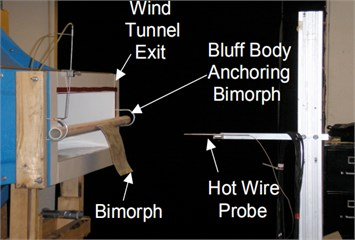Fig. 5. Piezoelectric array energy harvester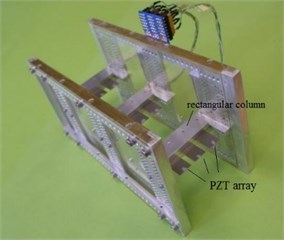Fig. 6. Piezoelectric energy harvester of rubber rod cylinder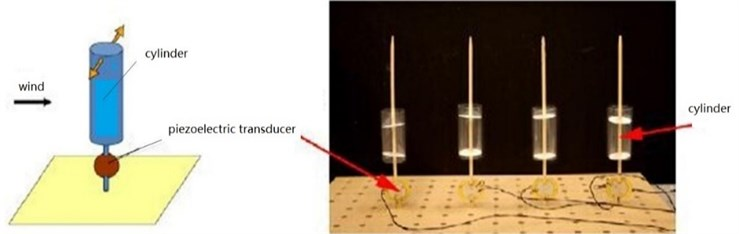Another type is using the vortex-induced vibration of the blunt body when it is supported by elastic support [15, 16]. Hobbs presented a piezoelectric energy harvester based on vortex-induced vibration of the rubber rod cylinder , shown in Fig. 6. The cylinder structure is supported by the elastic rubber rod and the piezoelectric transducer is installed at the root of the rubber rod where the strain is maximum. With the wind speed of 3 m/s, the output power of 96 μW was generated. Dai compared four distinct vortex-induced vibrations piezoelectric energy harvesters by experiment . The difference between these energy harvesters is the installation of the cylindrical bluff body at the tip of cantilever beam with different orientations (bottom, top, horizontal, and vertical). The results show that the bluff body should be aligned with the beam for low wind speeds (< 2 m/s) and perpendicular to the beam at high wind speeds (> 2 m/s) to design efficient energy harvesters.

Table 2. Parameters of piezoelectric energy harvester with vortex-induced vibration type

 Researcher Airdamper Piezoelectricmaterial Vibration frequency(Hz) Airflow velocity(m/s) Outputpower Matching resistance (KΩ) Powerdensity(mw/cm3) Robbins CylinderBluff body PVDFcantilever 5 6.7 10 mW 250 4.3 Akaydin CylinderBluff body PVDFcantilever 48.4 7.23 4 μW 1000 0.3 Pobering Rectangular bluff body PVDFcantilever 600 45 0.1 mW 6.4 2 Nguyen Triangular bluff body PVDFcantilever 876 20.7 0.59 nW 310 0.32 Weinstein CylinderBluff body PZTcantilever 40 5 3 mW 220 0.2 Hobbs Rubber rodBluff body PZTcantilever 20 3 96 μW 1000 2.4 H. L. Dai Cylindrical bluff body PZTcantilever 4 22 1.2 mW 2460 2.22

PVDF or PZT behind the blunt body can be formed as splitter plate, which can suppress the occurrence of vortex-induced vibration [19, 20]. When the inertia and stiffness of the splitter plate are low enough, this suppression will be weakened. As a result, PZT beams with high power density and high stiffness will suppress vortex-induced vibrations, while PVDF beams with low power density and high flexibility are prone to vortex-induced vibrations, which both can lead to low power density of the energy harvester.

#### 3.3. Piezoelectric energy harvester with flutter type

Flutter is the self-excited vibration of elastic structure in uniform air due to the coupling of aerodynamic, elastic and inertia force. It is usually triggered when the wind speed is greater than the critical value. In other words, flexible structures have low critical value of the wind speed and are easy to happen flutter, while rigid structures are the opposite.

Parameters of piezoelectric energy harvester with flutter type are shown in Table 3. By simulating the way that the leaves sway in the wind to make the branches vibrate, Shuguang Li proposed a bioinspired piezo-leaf  and fabricated prototypes using flexible piezoelectric materials as stalks and polymer film as leaves, shown in Fig. 7. A peak output power of approximately 0.6 mW and maximum power density of approximately 2 mW/cm3 from a single leaf can be generated by experiment. Bryant used a wing structure at the free end of the PZT piezoelectric cantilever beam to harvest wind energy . The flutter of the wing causes the piezoelectric beam vibration together. With the wind speed of 8 m/s, the output of 2.2 mW can be generated. Nishigaki manufactured and tested a flag-type piezoelectric wind energy harvester with different shapes of PVDF, such as rectangle, square and trapezoid . Zhao presented a wind-induced flutter piezoelectric energy harvester composed of a cantilever beam and a flexible piezoelectric plate at the end , shown in Fig. 8. It was found that the open circuit voltage of 16.4 V and the output power of 3.1 mW were generated with the wind speed of 12.2 m/s by test. Orrego studied the vibration and energy harvesting characteristics of the flag-type piezoelectric wind energy harvester . The influence of the fluid-structure coupling type, circuit load characteristics, flow field characteristic and electromechanical coupling factor on the power generation performance of the piezoelectric energy harvester were analyzed.

Fig. 7. A bioinspired piezo-leaf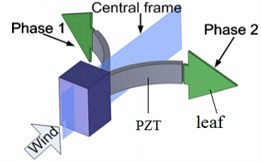a) Parallel-flow stalk-leaf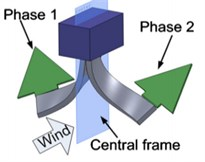b) Cross-flow stalk-leaf

Fig. 8. Flutter piezoelectric energy harvester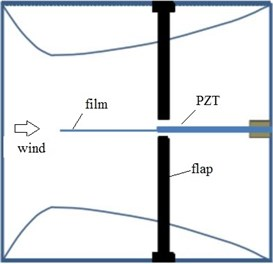From the above research, it can be found that due to the different piezoelectric materials of the transducing piezoelectric energy collector, the rigid structure has higher collection efficiency than the flexible structure, but the rigid structure has a larger size than the flexible structure. There is no universal theoretical formula for the determination of aerodynamic forces. The establishment of theoretical models, structural design and optimization are difficult. Research on rigid structures has progressed rapidly, and the lift, torque and attack of aerodynamic forces during the coupling process have been obtained. The empirical formula of angle can be expressed as :

(5)
${F}_{h}=\rho {V}^{2}b{C}_{l}\left({\alpha }_{eff}-{C}_{s}{\alpha }_{eff}^{3}\right),$
(6)
${M}_{a}=\rho {V}^{2}{b}^{2}{C}_{m}\left({\alpha }_{eff}-{C}_{s}{\alpha }_{eff}^{3}\right),$
(7)
${\alpha }_{eff}=\theta +\frac{\stackrel{˙}{h}}{V}+\left(\frac{1}{2}-a\right)b\frac{\stackrel{˙}{\theta }}{V},$

where ${F}_{h}$ is the aerodynamic lift, ${M}_{a}$ is the torque, ${\alpha }_{eff}$ is the effective angle of attack, $\rho$ is the fluid density, $V$ is the flow speed, $b$ is the half-chord length, ${C}_{l}$ is the lift coefficient, ${C}_{m}$ is the torque coefficient, ${C}_{s}$ is the nonlinear parameter related to stall, $a$ is the offset of the elastic axis relative to the half-chord, $h$ is the lateral displacement, $\theta$ is the rotation angle.

The flutter type energy harvesters have strict requirements on the direction of incoming flow and the size of flow velocity, so the direction of incoming flow and a certain range of flow velocity must be determined to produce vibration energy. Although it can adjust the incoming stream, but only within a very small range.

Table 3. Parameters of piezoelectric energy harvester with flutter type

 Researcher Airdamper Piezoelectricmaterial Vibration frequency (Hz) Airflow velocity(m/s) Outputpower Matching resistance(KΩ) Powerdensity(mw/cm3) Shuguang Li Leaf PVDFcantilever – 4 0.6 mW 30000 2 Bryant Plate PZTcantilever 16 8 2.2 mW – 9.14 Nishigaki Plate PVDFcantilever – 15 1.6 μW 900 6.67 Zhao Film PZTcantilever – 12.2 3.1 mW 300 27.8 Orrego Flag PZTcantilever 9 9 3.5 mW 10000 5

#### 3.4. Piezoelectric energy harvester with resonant type

In order to improve the output power of energy harvesters under low wind speed or weak vibration source, the resonator is used to amplify the oscillation pressure induced by sound wave or airflow to effectively increase the excitation energy and the power density. The acoustic modal frequency of the resonant cavity is a very important parameter, which directly affects the power generation of the generator. Existing studies have shown that the size of the acoustic modal frequency in the resonant cavity is mainly related to the resonant cavity. The length, diameter and distance from the resonance cavity to the air outlet are related [27, 28], the existing empirical formula can be expressed as:

(8)
$f=\frac{c}{4\left(h+\alpha d\right)},$

where $c$ is the speed of sound, $h$ and $d$ are the depth and diameter of the cavity respectively, and $\alpha$ is a constant related to the experiment.

When a standing wave is formed inside the resonator, the longitudinal particle velocity $u\left(x,t\right)$ and acoustic pressure $p\left(x,t\right)$ at the $n$-th resonant mode are represented as sinusoidal functions :

(9)
$p\left(x,t\right)={p}_{0}\mathrm{s}\mathrm{i}\mathrm{n}\frac{\pi \left(2n-1\right)x}{2L}\mathrm{e}\mathrm{x}\mathrm{p}\left(i2\pi {f}_{n}t\right),$
(10)
$u\left(x,t\right)={u}_{0}\mathrm{c}\mathrm{o}\mathrm{s}\frac{\pi \left(2n-1\right)x}{2L}\mathrm{e}\mathrm{x}\mathrm{p}\left(i2\pi {f}_{n}t\right),$

where $L$ is the resonator length, $x$ is the longitudinal distance measured from the tube open inlet, ${f}_{n}$ is the natural resonant frequency, and $n$ is the mode number. The maximum magnitude of particle velocity ${u}_{0}$ occurs at the resonator open inlet while the acoustic pressure reaches the maximum magnitude ${p}_{0}$ at the closed end.

The typical application is to harvest acoustic energy by the resonator. Yang placed a PZT cantilever at the top of the Helmholtz resonator , and the output power of 0.137 to 1.43 mW were generated with the sound excitation of sound pressure level of 100 dB and frequency of 170-206 Hz. Li placed PZT array inside the quarter wavelength resonator . With the sound excitation of sound pressure level of 110 dB, the output voltage of 15.68 V and the output power of 12.7 mW were generated. Yuan used a helical shaped resonator to harvest sound energy [32, 33], the output power of 7.3 μW were generated with the sound excitation of sound pressure level of 100 dB and frequency of 175 Hz. Zhao used the resonator to harvest sound energy by a friction electric nanogenerator . Compared with the traditional Helmholtz resonator, the double-tube Helmholtz resonator is more efficient and the output voltage is increased by 83 %.

In addition, combined with the mechanism of hydrodynamic sound source, the resonator had been studied and applied in the technology of harvesting air kinetic energy, shown in Table 4. Shu Chang presented a piezoelectric wind energy harvester with a resonator based on vortex-induced vibration . It was found that the output power with a resonator is higher than that of the one without a resonator within the low wind speed range, and the energy harvesting efficiency is greatly improved. Hernandez placed a piezoelectric transducer of disc type at the end of the resonant tube and arranged a baffle at a suitable position on the wall of the main pipe , shown in Fig. 9. With the flow speed of 2.6 m/s, the pressure of 170 Pa, the frequency of 445 Hz and the maximum output power of 0.55 mW were generated. Sun Daming presented a mean flow acoustic engine capable of wind energy harvesting [37-40], stable standing wave acoustic fields were established in specific ranges of air flow velocity. With a mean flow velocity of 50.52 m/s and a mean pressure of 106.19 kPa, the maximum pressure amplitude of 6.2 kPa was achieved, which fully shows its application prospect in wind energy harvesting. Lei designed an airflow resonant piezoelectric generator with the diameter of 34mm and simply studied the characteristics of the generator . The experiment was measured that when the flow velocity of 300 m/s, the vibration frequency of 1.2 kHz, the generator output voltage of 39.5 V and the output power of 1.6 W were generated, which proved that the airflow resonant generator is suitable for low power fuze. Xu designed a fuze micro-electromechanical airflow excitation power supply based on the principle of piezoelectric power generator , which was verified to be feasible with the inlet airflow velocity of 50 m/s-300 m/s. Chen presented an airflow resonant piezoelectric generator with the annular gap structure [43-45], shown in figure 10. With the flow velocity of 159 m/s, the vibration frequency of the generator can be reached 6-7 kHz. And the open circuit voltage of 22 V, and the output power of 58 mW were generated with the matching resistance of 3 kΩ. Wen designed a vortex-induced vibration self generating device based on the principle of vortex-induced vibration and resonant acoustic amplification . The experimental results demonstrated that the self generating device can produce an open circuit voltage of 6.0 V with the wind speed of 5 m/s.

Under the action of pressure fluctuation, the standing wave resonant can be formed inside the resonator of the resonant piezoelectric energy harvester, which can generate stable high-frequency vibration to effectively improve the excitation energy and increase the power density of energy harvesting. So, the piezoelectric energy harvester with resonant types have potential application.

Fig. 9. Mean flow piezoelectric energy harvester with baffle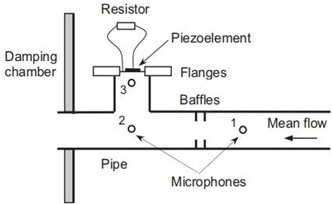a) The schematic diagram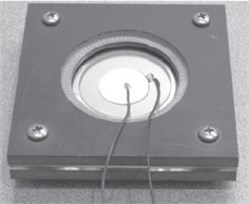b) The prototype

Fig. 10. Small airflow vibration piezoelectric generator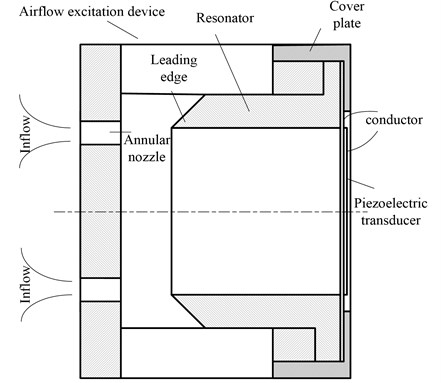Table 4. Parameters of piezoelectric energy harvester with resonant type

 Researcher Airdamper Piezoelectricmaterial Vibration frequency(Hz) Airflow velocity (m/s) Outputpower Matching resistance (kΩ) Power density(mw/cm3) Shu Chang Cylinder and resonator PZTcantilever – 12 2 V – – Hernandez Baffle and resonator PZT circularplate 445 2.6 0.55 mW 10 – Lei Junming Tip and resonator PZT circularplate 1200 300 1600 mW 1 440 Chen Hejuan Tip and resonator PZT circularplate 7000 159 85 mW 3 512 Wen Sheng Cylinder and resonator PVDFcantilever 110 5 6 V – –

#### 4. Conclusions

According to the different mechanisms of flue-induced vibration effect, the types and main parameters and indicators of the above different environmental airflow energy collectors are summarized in Table 5.

Table 5. Parameters of piezoelectric energy harvester with different types

 Type Piezoelectricmaterial Vibration frequency (Hz) Airflow velocity (m/s) Output power Power density (mw/cm3) Rotation PZTcantilever < 65 < 5 < 7.5 < 12.4 Vortex-induced vibration PVDF/PZTcantilever < 100 < 45 < 10 < 4.3 Flutter PVDF/PZTcantilever < 20 < 15 < 3.5 < 27.8 Resonant PZTmembrane < 10000 < 300 < 1600 < 512

After comparative analysis, it is summarized as follows:

1) The flow velocity is low. In addition to the energy harvesting of acoustic resonant type, the inflow velocity of other wind-induced vibration energy harvesters are generally lower than 45 m/s. There are few studies on energy harvesting within high velocity range.

2) The frequency is low. Except for the resonant type energy harvesting device, the wind-induced vibration energy is generally lower than 100 Hz. However, according to the empirical formula ${R}_{OP}=1/\omega {C}_{P}$, it can be seen that when the frequency increases, the internal resistance of the piezoelectric generator can be significantly reduced, which has a significant effect on the improvement of the output power. From the perspective of improving the energy conversion efficiency, the cavity type energy collection devices have great advantages.

3) Poor adaptability to working environment. Flutter and gallop type energy collection devices have strict requirements on the direction and velocity of the airflow. The direction of flow and a certain range of flow rate must be formulated to generate vibration energy. Although the resonant cavity type energy collector can perform inflow modulation, but it can only modulate the incoming flow within a certain range. How to make the energy harvester system oriented to the continuous and efficient energy output under the wide velocity range is the key problem faced by the energy harvester system based on flow-induced vibration.

4) Contradiction between volume and energy. When the single natural frequency of piezoelectric transducer cannot meet the demand for energy supply, the wideband frequency of piezoelectric transducer can capture more energy undoubtedly [47-49]. But it is at the cost of increasing the size and difficulty of the structure. To solve the contradiction between volume and energy caused by structure miniaturization, the cooperative energy exchange of multiple piezoelectric vibrators is an inevitable development trend.

#### Acknowledgements

The research is financially supported by the Jiangsu Colloge “Qing Lan Project” of China, the Jiangsu Colloge Natural Science Foundation of China, the Changzhou Applied Basic Research Plan of China (CJ20200010), the High-end Research Project of Jiangsu Vocational Professional Teacher of China (2020GRGDYX036).

1. Wang Shuo and Ding Lu, “Standardization situation and requirement of internet of things wireless senor networks,” (in Chinese), China Standardization, Vol. 2018, No. 21, pp. 69–73, 2018. [Search CrossRef]
2. Q. Zhou et al., “Transparent-flexible-multimodal triboelectric nanogenerators for mechanical energy harvesting and self-powered sensor applications,” Nano Energy, Vol. 48, pp. 471–480, Jun. 2018, https://doi.org/10.1016/j.nanoen.2018.03.074 [Publisher]
3. H. R. Foruzande, A. Hajnayeb, and A. Yaghootian, “Nanoscale piezoelectric vibration energy harvester design,” AIP Advances, Vol. 7, No. 9, p. 095122, Sep. 2017, https://doi.org/10.1063/1.4994577 [Publisher]
4. S. Priya, “Modeling of electric energy harvesting using piezoelectric windmill,” Applied Physics Letters, Vol. 87, No. 18, p. 184101, Oct. 2005, https://doi.org/10.1063/1.2119410 [Publisher]
5. S. Priya, C.-T. Chen, D. Fye, and J. Zahnd, “Piezoelectric windmill: a novel solution to remote sensing,” Japanese Journal of Applied Physics, Vol. 44, No. 3, pp. L104–L107, Jan. 2005, https://doi.org/10.1143/jjap.44.l104 [Publisher]
6. R. Myers, M. Vickers, H. Kim, and S. Priya, “Small scale windmill,” Applied Physics Letters, Vol. 90, No. 5, p. 054106, Jan. 2007, https://doi.org/10.1063/1.2435346 [Publisher]
7. Hyung-Kwan Chang, Daejoong Kim, and Jungyul Park, “Design and analysis of portable loadless wind power source for ubiquitous sensor network,” in 2nd International Conference on Computer and Automation Engineering (ICCAE 2010), pp. 93–96, Feb. 2010, https://doi.org/10.1109/iccae.2010.5451509 [Publisher]
8. C. H. Yang et al., “A high efficient piezoelectric windmill using magnetic force for low wind speed in wireless sensor networks,” Journal of the Korean Physical Society, Vol. 73, No. 12, pp. 1889–1894, Dec. 2018, https://doi.org/10.3938/jkps.73.1889 [Publisher]
9. Kan Junwu and Lv Peng, “Performance analysis and test of vortex induced vibration piezoelectric wind harvester,” (in Chinese), Transactions of the Chinese Society for Agricultural Machinery, Vol. 52, No. 4, pp. 411–417, 2021. [Search CrossRef]
10. W. P. Robbins, D. Morris, I. Marusic, and T. O. Novak, “Wind-generated electrical energy using flexible piezoelectric materials,” in ASME 2006 International Mechanical Engineering Congress and Exposition, pp. 1–9, Jan. 2006, https://doi.org/10.1115/imece2006-14050 [Publisher]
11. H. D. Akaydın, N. Elvin, and Y. Andreopoulos, “Wake of a cylinder: a paradigm for energy harvesting with piezoelectric materials,” Experiments in Fluids, Vol. 49, No. 1, pp. 291–304, Jul. 2010, https://doi.org/10.1007/s00348-010-0871-7 [Publisher]
12. S. Pobering, S. Ebermeyer, and N. Schwesinger, “Generation of electrical energy using short piezoelectric cantilevers in flowing media,” in SPIE Smart Structures and Materials + Nondestructive Evaluation and Health Monitoring, Mar. 2009, https://doi.org/10.1117/12.815189 [Publisher]
13. H.-D. Tam Nguyen, H.-T. Pham, and D.-A. Wang, “A miniature pneumatic energy generator using Kármán vortex street,” Journal of Wind Engineering and Industrial Aerodynamics, Vol. 116, pp. 40–48, May 2013, https://doi.org/10.1016/j.jweia.2013.03.002 [Publisher]
14. L. A. Weinstein, M. R. Cacan, P. M. So, and P. K. Wright, “Vortex shedding induced energy harvesting from piezoelectric materials in heating, ventilation and air conditioning flows,” Smart Materials and Structures, Vol. 21, No. 4, p. 045003, Apr. 2012, https://doi.org/10.1088/0964-1726/21/4/045003 [Publisher]
15. R. Naseer, H. L. Dai, A. Abdelkefi, and L. Wang, “Piezomagnetoelastic energy harvesting from vortex-induced vibrations using monostable characteristics,” Applied Energy, Vol. 203, pp. 142–153, Oct. 2017, https://doi.org/10.1016/j.apenergy.2017.06.018 [Publisher]
16. L. B. Zhang, A. Abdelkefi, H. L. Dai, R. Naseer, and L. Wang, “Design and experimental analysis of broadband energy harvesting from vortex-induced vibrations,” Journal of Sound and Vibration, Vol. 408, pp. 210–219, Nov. 2017, https://doi.org/10.1016/j.jsv.2017.07.029 [Publisher]
17. W. B. Hobbs, Piezoelectric Energy Harvesting: Vortex Induced Vibrations in Plants, Soap Films, and Arrays of Cylinders. Atlanta, USA: Georgia Institute of Technology, 2010. [Search CrossRef]
18. H. L. Dai, A. Abdelkefi, Y. Yang, and L. Wang, “Orientation of bluff body for designing efficient energy harvesters from vortex-induced vibrations,” Applied Physics Letters, Vol. 108, No. 5, p. 053902, Feb. 2016, https://doi.org/10.1063/1.4941546 [Publisher]
19. Kan Jun-Wu et al., “Research status and prospect of vortex-induced vibration micro-fluid energy harvester,” (in Chinese), Optics and Precision Engineering, Vol. 25, No. 6, pp. 1502–1512, 2017, https://doi.org/10.3788/ope.20172506.1502 [Publisher]
20. R. Song, X. Shan, F. Lv, and T. Xie, “A study of vortex-induced energy harvesting from water using PZT piezoelectric cantilever with cylindrical extension,” Ceramics International, Vol. 41, pp. S768–S773, Jul. 2015, https://doi.org/10.1016/j.ceramint.2015.03.262 [Publisher]
21. S. Li, J. Yuan, and H. Lipson, “Ambient wind energy harvesting using cross-flow fluttering,” Journal of Applied Physics, Vol. 109, No. 2, p. 026104, Jan. 2011, https://doi.org/10.1063/1.3525045 [Publisher]
22. M. Bryant and E. Garcia, “Modeling and testing of a novel aeroelastic flutter energy harvester,” Journal of Vibration and Acoustics, Vol. 133, No. 1, Feb. 2011, https://doi.org/10.1115/1.4002788 [Publisher]
23. T. Nishigaki, “Development of a piezoelectric polymer energy harvesting flag,” SPIE Smart Structures and Materials + Nondestructive Evaluation and Health Monitoring, Apr. 2013, https://doi.org/10.1117/12.2012512 [Publisher]
24. Zhao Xingqiang, Basic Theory and Key Technologies of Micro Piezoelectric Wind-Induced-Vibration Energy Harvester Based on Flutter, (in Chinese). Chongqing: Chongqing Universty, 2013. [Search CrossRef]
25. S. Orrego et al., “Harvesting ambient wind energy with an inverted piezoelectric flag,” Applied Energy, Vol. 194, pp. 212–222, May 2017, https://doi.org/10.1016/j.apenergy.2017.03.016 [Publisher]
26. Zhao Xingqiang, Wang Junlei, and Cai Jun, “Research status of miniature wind energy collector based on wind-induced vibration effect,” (in Chinese), Vibration and Shock, Vol. 16, pp. 32–43, 2017. [Search CrossRef]
27. J. Kastner and M. Samimy, “Development and characterization of hartmann tube fluidic actuators for high-speed flow control,” AIAA Journal, Vol. 40, No. 10, pp. 1926–1934, Oct. 2002, https://doi.org/10.2514/2.1541 [Publisher]
28. Zhang Lijie, Tian Zhangfu, and Zeng Xinwu, “Analysis of determinants of resonance frequency of Hartmann resonance,” (in Chinese), Acoustic Technology, Vol. 27, No. 5, pp. 212–213, 2008. [Publisher]
29. H. Zou, Q. Shi, H. Cai, and J. Liu, “The multi-harmonic excitation characteristic of airflow piezoelectric generator,” Journal of Vibroengineering, Vol. 23, No. 5, pp. 1219–1229, Aug. 2021, https://doi.org/10.21595/jve.2021.21863 [Publisher]
30. J. Yang, J. Chen, Y. Liu, W. Yang, Y. Su, and Z. L. Wang, “Triboelectrification-based organic film nanogenerator for acoustic energy harvesting and self-powered active acoustic sensing,” ACS Nano, Vol. 8, No. 3, pp. 2649–2657, Mar. 2014, https://doi.org/10.1021/nn4063616 [Publisher]
31. B. Li, A. J. Laviage, J. H. You, and Y.-J. Kim, “Harvesting low-frequency acoustic energy using quarter-wavelength straight-tube acoustic resonator,” Applied Acoustics, Vol. 74, No. 11, pp. 1271–1278, Nov. 2013, https://doi.org/10.1016/j.apacoust.2013.04.015 [Publisher]
32. M. Yuan, Z. Cao, J. Luo, J. Zhang, and C. Chang, “An efficient low-frequency acoustic energy harvester,” Sensors and Actuators A: Physical, Vol. 264, pp. 84–89, Sep. 2017, https://doi.org/10.1016/j.sna.2017.07.051 [Publisher]
33. M. Yuan, X. Wang, and Z. Ding, “Low frequency acoustic energy harvesting adopting slit Helmholtz resonator,” Vibroengineering Procedia, Vol. 20, pp. 151–155, Oct. 2018, https://doi.org/10.21595/vp.2018.20245 [Publisher]
34. H. Zhao et al., “Dual‐tube Helmholtz resonator‐based triboelectric nanogenerator for highly efficient harvesting of acoustic energy,” Advanced Energy Materials, Vol. 9, No. 46, p. 1902824, Dec. 2019, https://doi.org/10.1002/aenm.201902824 [Publisher]
35. Shu Chang, Zhang Jiantao, and Wu Song, “Piezoelectric wind energy harvester with resonant cavity,” (in Chinese), Journal of Vibration and Shock, Vol. 37, No. 3, pp. 22–26, 2018. [Search CrossRef]
36. R. Hernandez, S. Jung, and K. I. Matveev, “Acoustic energy harvesting from vortex-induced tonal sound in a baffled pipe,” Proceedings of the Institution of Mechanical Engineers, Part C: Journal of Mechanical Engineering Science, Vol. 225, No. 8, pp. 1847–1850, Aug. 2011, https://doi.org/10.1177/0954406211406017 [Publisher]
37. Y. Yu et al., “CFD study on mean flow engine for wind power exploitation,” Energy Conversion and Management, Vol. 52, No. 6, pp. 2355–2359, Jun. 2011, https://doi.org/10.1016/j.enconman.2010.12.046 [Publisher]
38. Yan Yu, Daming Sun, Ya Xu, Haijun Chen, and Limin Qiu, “Numerical study on mean flow-induced acoustic oscillation,” (in Chinese), Journal of Engineering Thermophysics, Vol. 33, No. 1, pp. 23–26, Nov. 2012. [Search CrossRef]
39. Xu Ya and Sun Daming, “Experimental study on acoustic characteristics of mean flow acoustic engine,” (in Chinese), Journal of Engineering Thermophysics, Vol. 33, No. 12, pp. 2034–2037, 2012. [Search CrossRef]
40. D. Sun, Y. Xu, H. Chen, K. Wu, K. Liu, and Y. Yu, “A mean flow acoustic engine capable of wind energy harvesting,” Energy Conversion and Management, Vol. 63, pp. 101–105, Nov. 2012, https://doi.org/10.1016/j.enconman.2011.12.035 [Publisher]
41. Lei Junming, “Fuze airflow resonance piezoelectric generator,” (in Chinese), Journal of Detection and Control, Vol. 31, No. 1, pp. 23–26, 2009. [Search CrossRef]
42. Xu Wei, Design and Research of Fuze Micro-Electromechanical Airflow Excitation Power Supply Based on Principle of Piezoelectric Power Generation, (in Chinese). Nanjing University of Science and Technology, 2012. [Search CrossRef]
43. H. Zou, H. Chen, and X. Zhu, “Piezoelectric energy harvesting from vibrations induced by jet-resonator system,” Mechatronics, Vol. 26, pp. 29–35, Mar. 2015, https://doi.org/10.1016/j.mechatronics.2015.01.002 [Publisher]
44. Zou Huajie, Zhang Bo, Chen Hejuan, Zhang Jianghua, and Wang Zeping, “Experimental study on small airflow vibration piezoelectric generator for fuze,” (in Chinese), Journal of Vibration and Shock, Vol. 37, No. 12, pp. 35–40, 2018. [Search CrossRef]
45. Z. Li, J. Li, and H. Chen, “Experimental research on excitation condition and performance of airflow-induced acoustic piezoelectric generator,” Micromachines, Vol. 11, No. 10, p. 913, Sep. 2020, https://doi.org/10.3390/mi11100913 [Publisher]
46. Wen Sheng, Li Shenghua, and Zhang Jiantao, “Design and experiment of vortex-induced vibration self-generating device based on principle of resonant acoustic amplification,” (in Chinese), Agricultural Machinery, Vol. 48, No. 11, pp. 204–214, 2017. [Search CrossRef]
47. Liu X. J. and Chen R. W., “Analysis on output power for multi-direction piezoelectric vibration energy harvester,” Transactions of Nanjing University of Aeronantics and Astronantics, Vol. 31, No. 6, pp. 668–675, 2014. [Search CrossRef]
48. Q. Tang and X. Li, “Two-stage wideband energy harvester driven by multimode coupled vibration,” IEEE/ASME Transactions on Mechatronics, Vol. 20, No. 1, pp. 115–121, Feb. 2015, https://doi.org/10.1109/tmech.2013.2296776 [Publisher]
49. Deng Zhiqiang and Wang Xiang, “Design and experiment of wideband piezoelectric vibration energy harvester,” (in Chinese), Journal of Zhejiang University, Vol. 51, No. 11, pp. 2287–2291, 2017. [Search CrossRef]

#### Cited By

 Energies Jiatong Chen, Bin Bao, Jinlong Liu, Yufei Wu, Quan Wang 2022